### IMO Shortlist 2010 problem G5

Kvaliteta:
Avg: 0,0
Težina:
Avg: 8,0
Let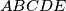$ABCDE$ be a convex pentagon such that$BC \parallel AE,$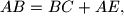$AB = BC + AE,$ and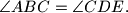$\angle ABC = \angle CDE.$ Let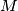$M$ be the midpoint of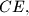$CE,$ and let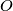$O$ be the circumcenter of triangle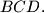$BCD.$ Given that$\angle DMO = 90^{\circ},$ prove that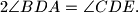$2 \angle BDA = \angle CDE.$

Proposed by Nazar Serdyuk, Ukraine
Izvor: Međunarodna matematička olimpijada, shortlist 2010